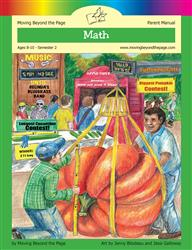Age 5-7 Reading Is Now Available!

# Common Core Alignment

CCSS.Math.Content.4.NF.3.b - Decompose a fraction into a sum of fractions with the same denominator in more than one way, recording each decomposition by an equation. Justify decompositions, e.g., by using a visual fraction model. Examples: 3/8 = 1/8 + 1/8 + 1/8 ; 3/8 = 1/8 + 2/8 ; 2 1/8 = 1 + 1 + 1/8 = 8/8 + 8/8 + 1/8.

## 5: MathUnit 5: Fractions
Lesson 5: Building Fractions